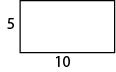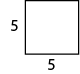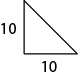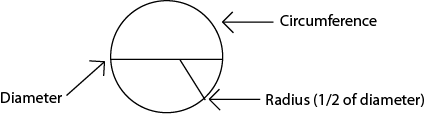First Line Sales
PO Box 200
Vernon, VT 05354

www.firstlinesales.com

Construction Estimating Formulas & Useful Information

Formulas, equations, tables, facts, specifications and other
information useful in planning and estimating construction,
decorating and other projects.

2.  The area of a rectangle is equal to its base x height. (A = b x h).For example:     10 x 5 = 50

3.  The area of a square is equal to its base x height (see above).
-or-
The area of a square is equal to the square of one of its sides. (A = S squared).For example:     5²=25

4.  The area of a triangle is equal to 1/2 the product of its base and height. A = 1/2 (b x h).For example:     10 x 10 = 100 x 1/2 = 50

5.  CirclesThe area of a circle is equal to 1/2 the product of its radius and circumference. (A = 1/2 (r x c).

For example:            radius = 5, circumference = 31.42:   5 x 31.42 = 157.1 x 1/2 = 78.55

-or-

A=π r², 3.1416(5 x 5) = 3.1416 x 25 = 78.54

-or-

A= d² x .7854

Find the circumference of a circle by multiplying the diameter x (π).  Find the volume of a cylinder by multiplying the height x the area of the circle.

*π = 3.1416

This information is believed to be accurate.  The Polymer Roof Factory or First Line Sales will not be liable for errors and omissions.  Specifications, detail, and other information should be verified by the user.